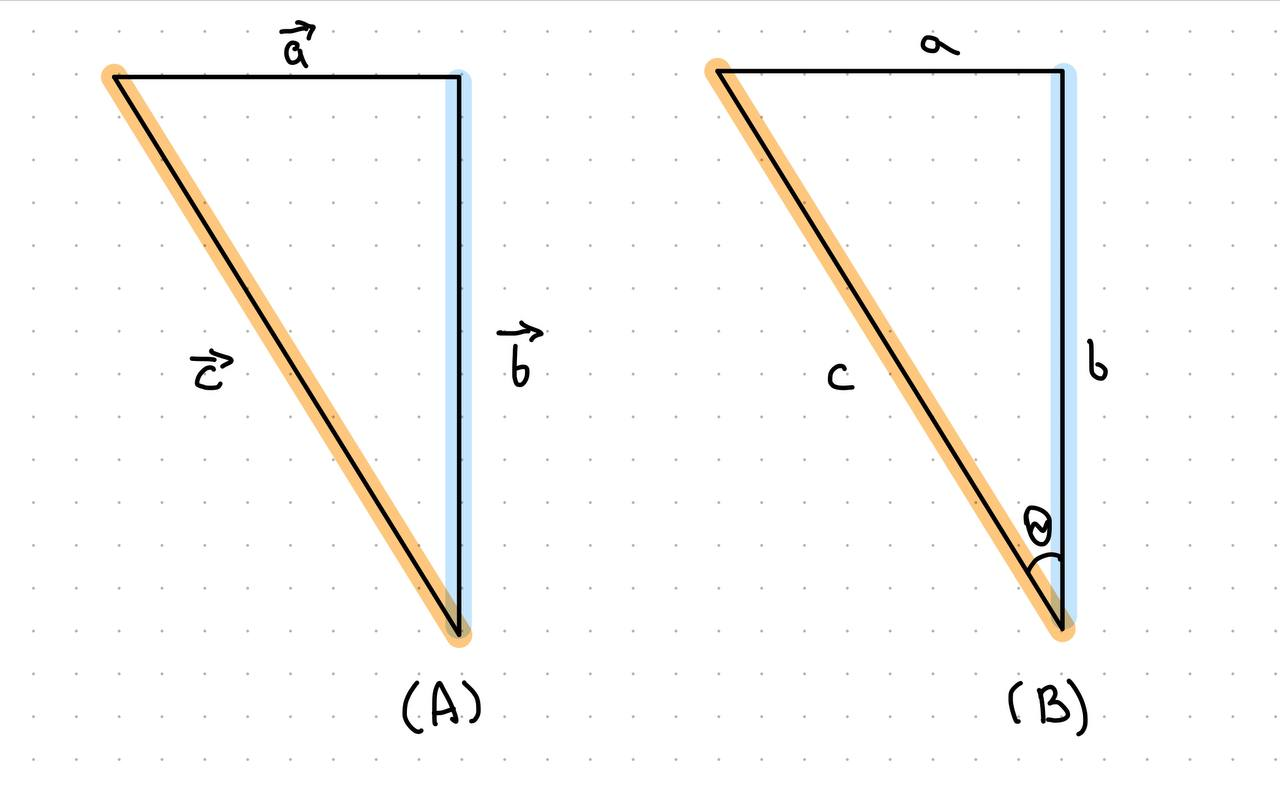Grimanijd

2022-07-05

Finding length or magnitude using vector addition and the Pythagorean theorem.
I am trying to understand why vector addition and the Pythagorean theorem are giving different results?According to diagram (A) : $\stackrel{\to }{a}+\stackrel{\to }{b}=\stackrel{\to }{c}$now suppose : magnitude of $\stackrel{\to }{a}=3$, magnitude of $\stackrel{\to }{b}=4$ then
$‖\stackrel{\to }{c}‖=‖\stackrel{\to }{a}‖+‖\stackrel{\to }{b}‖$
$‖\stackrel{\to }{c}‖=3+4$
magnitude of c = 7
Pythagorean Theorem
Now when we consider this as a triangle shown in diagram (B)
Similarly, length of a=3, length of b=4
so according to Pythagorean theorem
${\text{hypotenuse}}^{2}={\text{opposite}}^{2}+{\text{adjacent}}^{2}$
${c}^{2}={a}^{2}+{b}^{2}$i.e.
${c}^{2}={3}^{2}+{4}^{2}$
$c=\sqrt{9+16}=\sqrt{25}$
length of c = 5
Why there is inconsistency in the results? I am doing something wrong?
Thanks!Jamiya Costa

Expert

The magnitude of a vector $\left(\stackrel{\to }{a}+\stackrel{\to }{b}\right)$ is obtained in a similar way to that for Pythagorean triangles. It is incorrect to simply add up magnitudes of $\stackrel{\to }{a}$ and $\stackrel{\to }{b}$ .
Rather,$‖\stackrel{\to }{c}‖=\sqrt{‖\stackrel{\to }{a}‖+‖\stackrel{\to }{b}‖}$

Do you have a similar question?